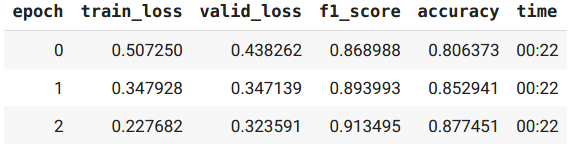# [Solved] How to apply layer-wise learning rates (discriminative lr)?

Hi all, How can I make this work ?

I have

``````len(hf_electra_param_splitter(model)) == 14
lrs = get_layer_lrs(...)
len(lrs) == 14
``````

I did

``````learn = Learner(
...
splitter=hf_electra_param_splitter,
lr=lrs,
)
learn = learn.fit_one_cycle(n_epoch=3)
``````

and get

``````/content/fastai2/fastai2/optimizer.py in set_hypers(self, **kwargs)
32
33     def unfreeze(self): self.freeze_to(0)
---> 34     def set_hypers(self, **kwargs): L(kwargs.items()).starmap(self.set_hyper)
35     def _set_hyper(self, k, v):
36         for v_,h in zip(v, self.hypers): h[k] = v_

....

/content/fastai2/fastai2/optimizer.py in set_hyper(self, k, v)
42         v = L(v, use_list=None)
43         if len(v)==1: v = v*len(self.param_lists)
---> 44         assert len(v) == len(self.hypers), f"Trying to set {len(v)} values for {k} but there are {len(self.param_lists)} parameter groups."
45         self._set_hyper(k, v)
46

AssertionError: Trying to set 14 values for lr but there are 1 parameter groups.
``````

I’d first check your number of layer groups after splitting. The quick way is to do a `learn.freeze()` followed by `.summary()` and see how many are frozen. But how are you defining your split? IE what does it look like?

I’d like to have look at your `splitter` function logic. Have you mapped them to params like so

``````L([...],[...],[...]).map(params)
``````

Thanks for your replies, here is my src code

``````# Names come from, for nm in model.named_modules(): print(nm)
def hf_electra_param_splitter(model, num_hidden_layers):
names = ['0.model.embeddings', *[f'0.model.encoder.layer.{i}' for i in range(num_hidden_layers)], '1']
groups = [ mod.parameters() for name, mod in model.named_modules() if name in names]
return groups

def get_layer_lrs(lr, lr_decay, num_hidden_layers):
# I think input layer as bottom and output layer as top, which is different from official repo
return [ lr * (lr_decay ** depth) for depth in reversed(range(num_hidden_layers+2))]
``````
``````learn = Learner(glue_dls['mrpc'], single_task_model,
loss_func=CrossEntropyLossFlat(),
metrics=[F1Score(), Accuracy()],
splitter=partial(hf_electra_param_splitter, num_hidden_layers=base_model.config.num_hidden_layers),
lr=get_layer_lrs(3e-4,0.8,base_model.config.num_hidden_layers),
).to_fp16()
learn.fit_one_cycle(n_epoch=3)
``````

``````ps = hf_electra_param_splitter(single_task_model,12)
print([ type(p) for p in ps])
print(len(ps))
lrs = get_layer_lrs(3e-4, 0.8, 12)
print(L(lrs))
``````
``````[<class 'generator'>, <class 'generator'>, <class 'generator'>, <class 'generator'>, <class 'generator'>, <class 'generator'>, <class 'generator'>, <class 'generator'>, <class 'generator'>, <class 'generator'>, <class 'generator'>, <class 'generator'>, <class 'generator'>, <class 'generator'>]
14
(#14) [1.649267441664001e-05,2.061584302080001e-05,2.5769803776000012e-05,3.221225472000001e-05,4.026531840000002e-05,5.033164800000002e-05,6.291456000000001e-05,7.864320000000003e-05,9.830400000000001e-05,0.00012288000000000002...]
``````

I suppose you should have 14 lists instead of just one. Try doing

``````groups = [[mod.parameters()] for name, mod in model.named_modules() if name in names]
``````

If you want to have exact one layer in each layer group

I try the change to get 14 lists instead of generators.
But I get another message.

``````---------------------------------------------------------------------------

AttributeError                            Traceback (most recent call last)

<ipython-input-32-b586e752124c> in <module>()

...

/content/fastai2/fastai2/optimizer.py in step(self)
80
81     def step(self):
---> 82         for p,pg,state,hyper in self.all_params(with_grad=True):
83             for cb in self.cbs: state = _update(state, cb(p, **{**state, **hyper}))
84             self.state[p] = state

15         res = L((p,pg,self.state[p],hyper) for pg,hyper in zip(self.param_lists[n],self.hypers[n]) for p in pg)
---> 16         return L(o for o in res if o.grad is not None) if with_grad else res
17

...

/content/fastai2/fastai2/optimizer.py in <genexpr>(.0)
15         res = L((p,pg,self.state[p],hyper) for pg,hyper in zip(self.param_lists[n],self.hypers[n]) for p in pg)
---> 16         return L(o for o in res if o.grad is not None) if with_grad else res
17

AttributeError: 'generator' object has no attribute 'grad'
``````

Hi, thanks to @kshitijpatil09 's advice,
I tried

``````groups = [ list(mod.parameters()) for name, mod in model.named_modules() if name in names]
``````

and I gothigher than not using discriminative lr

So much thank you !!4 Likes